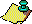Contents Next: Applications of the Up: Recognizing Numerical Previous: Introduction

# The PSLQ Integer Relation Algorithm

2. The PSLQ Integer Relation Algorithm In 1991 a new algorithm, known as ``PSLQ'' algorithm, was developed by Ferguson . It appears to combine some of the best features separately possessed by previous algorithms, including fast run times, numerical stability, numerical efficiency (i.e. successfully recovering a relation when the input is known to only limited precision), and a guaranteed completion in a polynomially bounded number of iterations. More recently a much simpler formulation of this algorithm was developed, and it has been extended to the complex number field . This newer, simpler version of PSLQ can be stated as follows: Let x be the n-long input real vector, and let nint denote the nearest integer function (for exact half-integer values, define nint to be the integer with greater absolute value). Let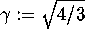. Then perform the following:

Initialize:

1. Set the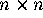matrices A and B to the identity.

2. For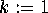to n: Set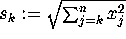; endfor. Set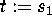. Forto n: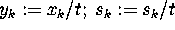; endfor.

3. Compute the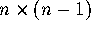matrix H as follows:

4. Perform full reduction on H, simultaneously updating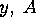and B:

Repeat until precision is exhausted or a relation has been detected:

1. Select m such that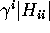is maximal when i = m.

2. Exchange entries m and m + 1 of y, corresponding rows of A and H, and corresponding columns of B.

3. If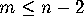then update H as follows:

4. Perform block reduction on H, simultaneously updatingand B:

5. Norm bound: Compute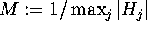, where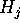denotes the j-th row of H. Then there can exist no relation vector whose Euclidean norm is less than M.

6. Termination test: If the largest entry of A exceeds the level of numeric precision used, then precision is exhausted. If the smallest entry of the y vector is less than the detection threshold, a relation has been detected and is given in the corresponding column of B.

With regards to the termination criteria in step 6, it sometimes happens that a relation is missed at the point of potential detection because the y entry is not quite as small as the detection threshold being used (the threshold is typically set to the ``epsilon'' of the precision level). When this happens, however, one will note that the ratio of the smallest and largest y vector entries is suddenly very small, provided sufficient numeric precision is being used. In a normal computer run using the PSLQ algorithm, prior to the detection of a relation, this ratio is seldom smaller than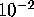. Thus if this ratio suddenly decreases to a very small value, such as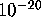, then almost certainly a relation has been detected --- one need only adjust the detection threshold for the algorithm to terminate properly and output the relation. When detection does occur, this ratio may be thought of as a ``confidence level'' of the detection.
As a general rule, one can expect to detect a relation of degree n, with coefficients of size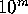, provided that the input vector is known to somewhat greater than m n digit precision, and provided that computations are performed using at least this level of numeric precision.Contents Next: Applications of the Up: No Title Previous: Introduction